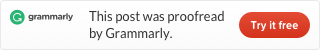# Leetcode 2 Add Two Numbers (Java)

Medium

You are given two non-empty linked lists representing two non-negative integers. The digits are stored in reverse order and each of their nodes contains a single digit. Add the two numbers and return it as a linked list.
You may assume the two numbers do not contain any leading zero, except the number 0 itself.

Example:
Input: (2 -> 4 -> 3) + (5 -> 6 -> 4)
Output: 7 -> 0 -> 8
Explanation: 342 + 465 = 807.

This is easy, we can just use the vertical addition method. Like this:

From the last digit of both numbers, one digit by one digit, add the digits. If the sum is smaller than 10, then put the sum on the result position. If the sum is larger or equals 10, put an additional flag on the higher position.

And this is the code:

Running time is 19ms, Time complexity is O(n), Space complexity is O(n).This site uses Akismet to reduce spam. Learn how your comment data is processed.